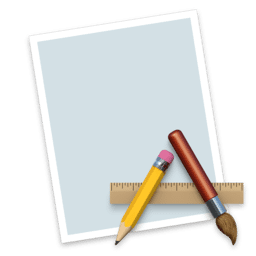Basic Shapes Formulas
1.0

0.0

Basic Shapes Formulas# Basic Shapes Formulas1.0

22 July 2016

28 different calculators that will simplify calculations.

## Overview

Basic Shapes Formulas comprises a selection of 28 different calculators that will simplify calculations. Complex calculations, such as Surface Area of Tetrahedron, Volume of Cylinder and others listed below will be handled easily with this app.

• Surface Area of Tetrahedron
• Total Surface Area of Frustum of Pyramid
• Volume of Frustum of Regular Pyramid
• Radius of a Circumscribed Circle
• Total Surface Area of a Right Circular Cylinder
• Lateral Surface Area of Frustum of Cone
• Height of a Frustum of a Right Circular Cone
• Surface Area of a Dodecahedron
• Total Surface Area of a Right Circular Cylinder
• Scale Factor of Frustum of Right Circular Cone
• Volume of Dodecahedron
• Volume of Cylinder
• Height of Right Circular Cone
• Area of Base of Right Circular Cone
• Total Surface Area of Right Circular Cone
• Volume of a Right Circular Cone
• Area of Spherical Lune Degrees
• Total Surface Area of Spherical Wedge Degrees
• Volume of Prolate Spheroid
• Volume of Spherical Wedge Radians
• Surface Area of a Prolate Spheroid
• Total Surface Area of Spherical Wedge Radians
• Area of Plane End Faces of a Right Circular Cylinder
• Surface Area of Icosahedron
• Scale Factor of Frustum of Pyramid based on Area
• Perimeter of Sector of Circle
• Volume of Tetrahedron
##### Features:
• Formula and description for each calculator
• Copy / Paste params and results
• Clean, simple and easy to use.

## What's new in Basic Shapes Formulas

Version 1.0:
• Initial release

#### Join over 500,000 subscribers.

Subscribe for our newsletter with best Mac offers from MacUpdate.

How would you rate Basic Shapes Formulas app?